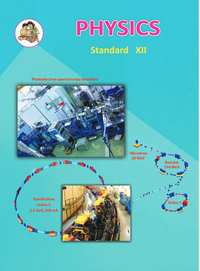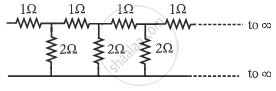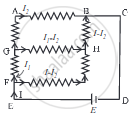# Balbharati solutions for Physics 12th Standard HSC Maharashtra State Board chapter 9 - Current Electricity [Latest edition]

#### Chapters## Chapter 9: Current Electricity

Exercises
Exercises [Pages 228 - 229]

### Balbharati solutions for Physics 12th Standard HSC Maharashtra State Board Chapter 9 Current ElectricityExercises [Pages 228 - 229]

Exercises | Q 1.1 | Page 228

Kirchhoff’s first law, i.e., ΣI = 0 at a junction, deals with the conservation of

• charge

• energy

• momentum

• mass

Exercises | Q 1.2 | Page 228

When the balance point is obtained in the potentiometer, a current is drawn from

• both the cells and auxiliary battery

• cell only

• auxiliary battery only

• neither cell nor auxiliary battery

Exercises | Q 1.3 | Page 228

In the following circuit diagram, an infinite series of resistances is shown. Equivalent resistance between points A and B is• infinite

• zero

• 2 Ω

• 30 Ω

Exercises | Q 1.4 | Page 228

Four resistances 10 Ω, 10 Ω, 10 Ωand 15 Ω form a Wheatstone’s network. What shunt is required across 15 Ωresistor to balance the bridge

• 10 Ω

• 15 Ω

• 20 Ω

• 30 Ω

Exercises | Q 1.5 | Page 228

A circular loop has a resistance of 40 Ω. Two points P and Q of the loop, which are one-quarter of the circumference apart are connected to a 24 V battery, having an internal resistance of 0.5 Ω. What is the current flowing through the battery?

• 0.5 A

• 1A

• 2A

• 3A

Exercises | Q 1.6 | Page 228
To find the resistance of a gold bangle, two diametrically opposite points of the bangle are connected to the two terminals of the left gap of a meter bridge. A resistance of 4 Ωis introduced in the right gap. What is the resistance of the bangle if the null point is at 20 cm from the left end?
• 2 Ω

• 4 Ω

• 8 Ω

• 16 Ω

Exercises | Q 2.01 | Page 228

Define or describe a Potentiometer.

Exercises | Q 2.02 | Page 228

Exercises | Q 2.03 | Page 228

Why should not the jockey be slided along the potentiometer wire?

Exercises | Q 2.04 | Page 228

Are Kirchhoff’s laws applicable to both AC and DC currents?

Exercises | Q 2.05 | Page 228

In Wheatstone’s meter-bridge experiment, the null point is obtained in the middle one-third portion of the wire. Why is it recommended?

Exercises | Q 2.06 | Page 228

State any two sources of errors in the meter-bridge experiment. Explain how they can be minimized.

Exercises | Q 2.07 | Page 228

What is a potential gradient? How is it measured? Explain.

Exercises | Q 2.08 | Page 228

On what factors does the potential gradient of the wire depend?

Exercises | Q 2.09 | Page 228

Why is a potentiometer preferred over a voltmeter for measuring emf?

Exercises | Q 2.1 | Page 228

State the uses of a potentiometer.

Exercises | Q 2.11 | Page 228

What are the disadvantages of a potentiometer?

Exercises | Q 2.12 | Page 228

Distinguish between a potentiometer and a voltmeter.

Exercises | Q 2.13 | Page 228

What will be the effect on the position of zero deflection if only the current flowing through the potentiometer wire is increased?

Exercises | Q 2.13 | Page 228

What will be the effect on the position of zero deflection if only the current flowing through the potentiometer wire is decreased?

Exercises | Q 3 | Page 228

With the help of a labelled diagram, show that the balancing condition of a Wheatstone bridge is

R_1/R_2 = R_3/R_4 where the terms have their usual meaning.

Exercises | Q 4 | Page 228

Explain with a neat circuit diagram how will you determine unknown resistance ‘X' by using meter bridge

Exercises | Q 5 | Page 228

Describe Kelvin’s method to determine the resistance of the galvanometer by using a meter bridge.

Exercises | Q 6 | Page 228

Describe how a potentiometer is used to compare the EMFs of two cells by connecting the cells individually.

Exercises | Q 7 | Page 229

Describe how a potentiometer is used to compare the emf's of two cells by the combination method.

Exercises | Q 8 | Page 229

Describe with the help of a neat circuit diagram how you will determine the internal resistance of a cell by using a potentiometer. Derive the necessary formula.

Exercises | Q 9 | Page 229

On what factors does the internal resistance of a cell depend?

Exercises | Q 10 | Page 229

A battery of emf 4 volt and internal resistance 1 Ω is connected in parallel with another battery of emf 1 V and internal resistance 1 Ω (with their like poles connected together). The combination is used to send current through an external resistance of 2 Ω. Calculate the current through the external resistance.

Exercises | Q 11 | Page 229

Two cells of emf 1.5 Volt and 2 Volt having respective internal resistances of 1 Ω and 2 Ω are connected in parallel so as to send current in the same direction through an external resistance of 5 Ω. Find the current through the external resistance.

Exercises | Q 12 | Page 229

A voltmeter has a resistance of 30 Ω. What will be its reading, when it is connected across a cell of emf 2 V having internal resistance 10 Ω?

Exercises | Q 13 | Page 229

A set of three coils having resistances 10 Ω, 12 Ω, and 15 Ω are connected in parallel. This combination is connected in series with a series combination of three coils of the same resistances. Calculate the total resistance and current through the circuit, if a battery of emf 4.1 Volt is used for drawing current.

Exercises | Q 14 | Page 229

A potentiometer wire has a length of 1.5 m and a resistance of 10 Ω. It is connected in series with the cell of emf 4 Volt and internal resistance 5 Ω. Calculate the potential drop per centimeter of the wire.

Exercises | Q 15 | Page 229

When two cells of emf's E1 and E2 are connected in series so as to assist each other, their balancing length on a potentiometer wire is found to be 2.7 m. When the cells are connected in series so as to oppose each other, the balancing length is found to be 0.3 m. Compare the emf's of the two cells.

Exercises | Q 16 | Page 229

The emf of a cell is balanced by a length of 120 cm of a potentiometer wire. When the cell is shunted by a resistance of 10 Ω, the balancing length is reduced by 20 cm. Find the internal resistance of the cell.

Exercises | Q 17 | Page 229

A potential drop per unit length along a wire is 5 × 10−3 V/m. If the emf of a cell balances against length 216 cm of this potentiometer wire, find the emf of the cell.

Exercises | Q 18 | Page 229

The resistance of a potentiometer wire is 8 Ω and its length is 8 m. A resistance box and a 2 V battery are connected in series with iL What should be the resistance in the box if it is desired to have a potential drop of 1 µV/mm?

Exercises | Q 19 | Page 229

Find the equivalent resistance between the terminals of A and B in the network shown in the figure below given that the resistance of each resistor is 10 ohm.Exercises | Q 20 | Page 229

A voltmeter has a resistance of 100 Ω. What will be its reading when it is connected across a cell of e.m.f. 2V and internal resistance 20Ω?

## Chapter 9: Current Electricity

Exercises## Balbharati solutions for Physics 12th Standard HSC Maharashtra State Board chapter 9 - Current Electricity

Balbharati solutions for Physics 12th Standard HSC Maharashtra State Board chapter 9 (Current Electricity) include all questions with solution and detail explanation. This will clear students doubts about any question and improve application skills while preparing for board exams. The detailed, step-by-step solutions will help you understand the concepts better and clear your confusions, if any. Shaalaa.com has the Maharashtra State Board Physics 12th Standard HSC Maharashtra State Board solutions in a manner that help students grasp basic concepts better and faster.

Further, we at Shaalaa.com provide such solutions so that students can prepare for written exams. Balbharati textbook solutions can be a core help for self-study and acts as a perfect self-help guidance for students.

Concepts covered in Physics 12th Standard HSC Maharashtra State Board chapter 9 Current Electricity are Current Electricity, Kirchhoff’s Laws of Electrical Network, Wheatstone Bridge, Potentiometer, Galvanometer.

Using Balbharati 12th Board Exam solutions Current Electricity exercise by students are an easy way to prepare for the exams, as they involve solutions arranged chapter-wise also page wise. The questions involved in Balbharati Solutions are important questions that can be asked in the final exam. Maximum students of Maharashtra State Board 12th Board Exam prefer Balbharati Textbook Solutions to score more in exam.

Get the free view of chapter 9 Current Electricity 12th Board Exam extra questions for Physics 12th Standard HSC Maharashtra State Board and can use Shaalaa.com to keep it handy for your exam preparation